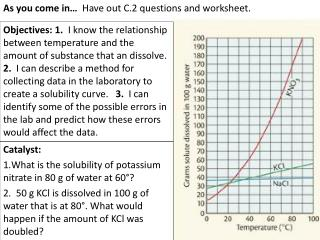DownloadDownload PresentationCatalyst: What is the solubility of potassium nitrate in 80 g of water at 60°?

# Catalyst: What is the solubility of potassium nitrate in 80 g of water at 60°?

Download Presentation## Catalyst: What is the solubility of potassium nitrate in 80 g of water at 60°?

- - - - - - - - - - - - - - - - - - - - - - - - - - - E N D - - - - - - - - - - - - - - - - - - - - - - - - - - -
##### Presentation Transcript

1. As you come in… Have out C.2 questions and worksheet. Objectives: 1. I know the relationship between temperature and the amount of substance that an dissolve. 2. I can describe a method for collecting data in the laboratory to create a solubility curve. 3. I can identify some of the possible errors in the lab and predict how these errors would affect the data. Catalyst: What is the solubility of potassium nitrate in 80 g of water at 60°? 50 g KCl is dissolved in 100 g of water that is at 80°. What would happen if the amount of KCl was doubled?

2. 3. Which substance is most soluble at 60° C? 5. What is the solubility of potassium nitrate at 90° C? 7. You have a solution of sodium nitrate containing 140 g at 65° C. Is the solution saturated, unsaturated, or supersaturated? 9. A solution of potassium iodide at 70° C contains 200 g of dissolved solute in 100 cm3 water. The solution is allowed to cool. At what new temperature would crystals begin to start forming?

3. 11. If a saturated solution of KNO3 at 20°C is heated to 80 °C, how much more KNO3 could be dissolved? 13. How much NaCl will dissolve in 350 g of water at 70 °C? 15. How much KNO3 will dissolve in 5.6 kg of water at 80 °C? 170-30=140g KNO3 170 40*3.5=140gNaCl 5.6 g * 1000g/1kg = 5600 g170/100 = ?/5600 g 170*5600/100= 9520 g KNO3 40 30

4. Solubility Lab Measure out exactly 4.00 grams of the salt (NH4Cl) and place in a large test tube. Add exactly 10 mL of distilled water to the test tube containing the salt. Place the thermometer in the tube (may be used to stir the solution) 4 g NH4Cl and 10 mL DI water 4 g NH4Cl

5. Using a hot water bath dissolve the salt. *Using TEST TUBE HOLDER* 4 g NH4Cl and 10 mL DI water water water clear water Hotplate Hotplate Hotplate

6. Constructing a Solubility Curve Data